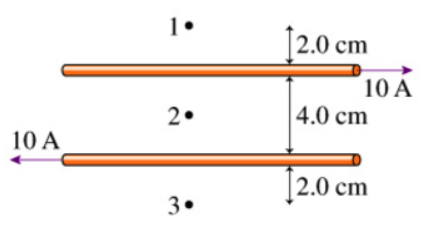# Problem: Part A. What is the magnetic field strength at point 1 in the figure? Express your answer using two significant figures.Part B. What is the magnetic field direction at point 1 in the figure?A. out of the figureB. into the page

###### FREE Expert Solution

The net magnetic field is given by:

Bnet = B1 - B2, where B1 is the magnetic field at point 1 and B2 is the magnetic field at point 2.

Magnetic field around a wire carrying current:

$\overline{){\mathbf{B}}{\mathbf{=}}\frac{{\mathbf{\mu }}_{\mathbf{0}}\mathbf{i}}{\mathbf{2}\mathbf{\pi }\mathbf{r}}}$

Part A

The magnetic field strength at point 1:

95% (3 ratings)###### Problem DetailsPart A. What is the magnetic field strength at point 1 in the figure? Express your answer using two significant figures.

Part B. What is the magnetic field direction at point 1 in the figure?

A. out of the figure

B. into the page